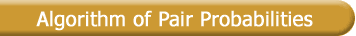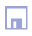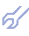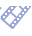At early stages of mathematical modeling scientists believed that for solution of interpolation problems probabilistic concept should be used : optimal value of output variable should correspond to maximum of a posteriori probability calculated by Bayes formula or to the rules of Walds' statistical decision theory. Soon it was established that we have not in our disposition initial data samplings sufficient for reliable calculation of empirical probabilities necessary for these calculations. Now we can say, that it was historically strategic mistake to refuse of probabilistic algorithms usage because except of usually used ordinary probabilities can be calculated pair probabilities which demands a very small number of data sampling lines for their calculation. Decision about optimal value of output variable should correspond to maximum of sum of ordinary and pairs empirical probabilities. To the number of ordinary random events E(y,xi) should be added the number of pairs random events E(y,xi,xj). The requirements to input data sample are decreased significantly: it is enough to have one observation for each pattern or discrete level of output variable. Algorithm is recommended for recognition of binary objects and for the veritability of input data control to avoid the possible experts errors in it.

Example: Recognition and forecast of situation with spring wheat crop.

The problem of wheat crop recognition and forecasting for field with constant cultivation order is solved. In series of years only fertilizations are changed. In table: X1 - length of bright day; X2 - contents of nitrogen in fertilization; X3 - contents of sodium; X4 - contents of potassium.

 Years (marked as letters) Factors Class of crop x1 x2 x3 x4 A 1 1 1 1 R1 ("Average") F 1 2 1 2 L 1 2 2 3 D 1 3 4 3 K 1 3 5 3 R2 ("Good") B 2 3 5 4 G 1 5 5 2 H 1 5 5 2 I 5 4 4 2 R3 ("Excellent") J 3 5 4 3 E 5 5 3 2 C 5 5 3 3 New 5 4 3 2 ?

Using this data sampling we calculate single and pair empirical probabilities. Sum of single empirical random events, proportional to ordinary empirical probabilities, are shown in table 2.

Table 2. Number of single probability events calculation.

 New-> 5 4 3 2 R1 0 1 3 4 Sum = 8 R2 6 1 3 3 Sum = 13 R3 6 3 4 2 Sum = 15 <-max

Sum of pairs of empirical random events are shown in table 3. There are shown all possible pairs of variables: their discrete values (called discreets) and sums of random events pairs.

Table 3. Sums of pair random events to reach possible discreets.

 xi - xj 5-4 5-3 5-2 4-3 4-2 3-2 Sum R1 0 0 0 3 4 12 19 R2 6 18 18 3 3 9 51 R3 18 24 12 12 6 8 80 <-max

Criterion for choice of next crop estimation: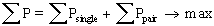where: Psingle = Esingle / N; Ppair = Epair / N; Epair(ij) = Esingle(i) * Esingle(j)
N - number of possible events.

For output vector X=(5,4,3,2) we found that crop will be "Excellent" (Sum=80).

Taking prehistory of process into account

Important moment is that discretization and normalizing according to Widrow rule (the same number of points for each level) can be applied to initial data. Forecast should be calculated for all levels of output variable Ri and the most probable levels should be chosen out as the most probable forecast for each given output vector of factors. To get high accuracy of forecast there is necessary to take into account random events calculated for each delay time separately. For example for Dollar rate forecast it is necessary to take into account several delayed values of Dollar rate. Criterion in following form would be calculated:Lead time of forecast can be estimated approximately by formulae:

T = h / RR

where: h - time step given in data sample, RR - error variance criterion (when RR = 0.01 lead time is about TL = 100 time steps).

When we have up to 100 factors in the sampling Combinatorial algorithm is recommended. When number of factors is equal from 100 to about 500 lines the multilayered GMDH algorithms can be used. In case when number of observations is big the algorithm of data sampling clusterization should be used, to transform initial data sampling into sampling of centers of clusters of physical clusterization coordinates.

Genetic types of algorithms, with crossover recombination of "mother" and "father" partial descriptions and random mutation of new models-candidates are recommended in cases when data sampling contains more than 500 variables. If we say that it is enough to have one line of data for each level of output variable in this algorithm it means according to genetic approach that we use algorithm of fern plants, having only "mother."